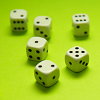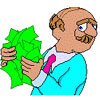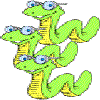#### You may also like### Roll These Dice

Roll two red dice and a green dice. Add the two numbers on the red dice and take away the number on the green. What are all the different possible answers?### Bipin's Choice

Bipin is in a game show and he has picked a red ball out of 10 balls. He wins a large sum of money, but can you use the information to decided what he should do next?### Trick or Treat

Mrs. Smith had emptied packets of chocolate-covered mice, plastic frogs and gummi-worms into a cauldron for treats. What treat is Trixie most likely to pick out?

# A Bit of a Dicey Problem

##### Age 7 to 11Challenge Level
William from Churchers College Junior School sent in his good explanations.

$1$a You can roll numbers between $2$ and $12$ with two six-sided dice
b $7$: Because you can roll combinations which use all the numbers on the dice.
$1-6$, $6-1$, $5-2$, $2-5$, $4-3$, $3-4$

$6$ combinations give $7$.
There are $36$ possible combinations when you threw two six-sided dice SO $6$ /$36 \times 100 = 16.67$ % chance of rolling $7$ with two six-sided dice.

$2$a With two ten-sided dice you can roll numbers between $2$ and $20$.
b  $11$; because there are ten combinations which give $11$ which use all the numbers on the dice
There are are $100$ possibilities when you roll two ten-sided dice.
So $10$/$100 \times 100 = 10$ % chance of rolling $11$ with $2$,  $10$ sided dice.

From Oscar at CCJS (which I think is Cheltenham College Junior School - but forgive me if I'm wrong!) also worked on this and sent in the following;

I wrote down every possible throw I could get using a black and white six-faced dice - it came to $36$ possibilites.
I counted how many of each total number I could get and found that the commonest total number to get was $7$
Two six-faced dice gave me $36$ possibilities. That is $6$ squared or $6 \times 6$.
The two six-faced dice had $7$ as the commonest number that we could get - that is, one greater than the number of sides on each dice.

Finally Tom from Fen Ditton C.P. School wrote to tell us what he already knew or what he found out by doing the activity:

$6$ numbered  dice: the most likely number is $7$ because is has got the most combinations.
$10$ numbered dice: $11$ is the most likely  because it has the most combinations.
The formula for this problem is:n $+1$     n= no. of  sides on the die.

Well done all of you, three excellent solutions sent in, keep up the good work!